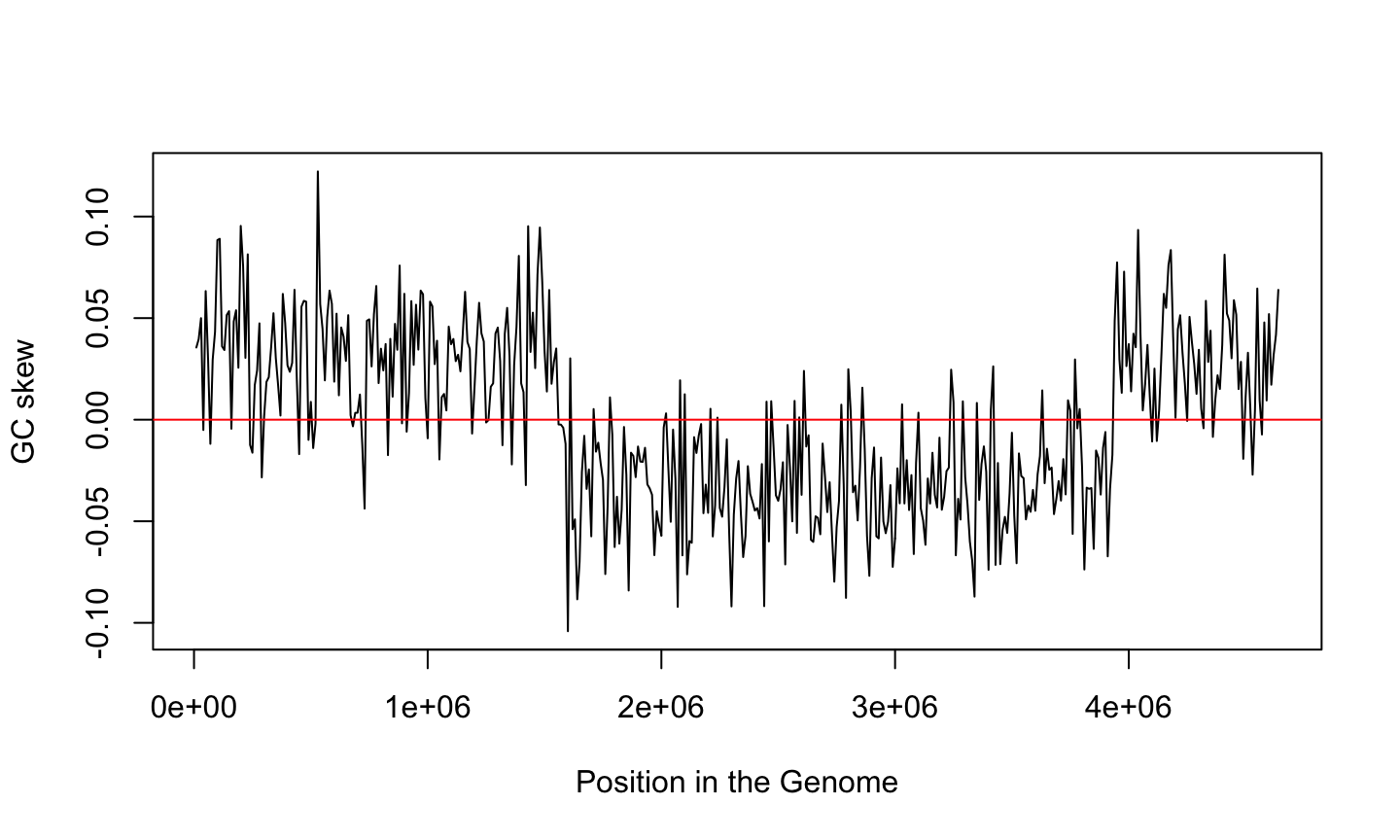March 8th, 2016

## Plan for Today

• Global v/s local genome statistics
• GC content
• GC skew
• What are they useful for
• How to calculate them
• function
• lapply/sapply
• cumsum

## What do we know?

• Some organisms have been sequenced
• We can read the sequences from FASTA files
• read.fasta returns a list of vectors of text
• We can create functions to do things with the sequence
• Functions are black boxes with inputs and outputs
• For example we do statistics of the sequence

## Why we do statistics on the sequence?

Statistics is a way to tell a story that makes sense of the data

In genomics, we look for biological sense

That story can be about the complete genome

• for example, the average GC content

or can be about some region of the genome

• for example, the GC skew on regions of size 10K

## What is the story told by GC skew?## Remember Chargaff second rule

For each DNA strand we have $$\%A \approx \%T$$ and $$\%G \approx \%C$$

This is because the substitution rate is presumably equal

Hence, the second parity rule only exists when there is no mutation or substitution

But the ratio of G over C is not uniform over the genome

Why?

## GC skew and genome replication

GC skew changes sign at the boundaries of the two replichores

This corresponds to DNA replication origin or terminus

The replication origin is usually called ori.

##How DNA replicates {.no-gap}

## How to calculate values on regions

All our calculations are done using functions

What should be

• the output?
• the input(s)?
• the name?

## Defining a GC skew function

Remember that an R function is defined like this

name <- function(input1, input2, ...) {
Calculate
return(output)
}

## The inputs

The GC skew result should depend on:

• the genomic sequence
• the start of the window
• the length of the window

These are the parameters of the function.
Do they have

• a name?
• a default value?

## The output

How do we transform the input parameters into the output value?

We can use any R function available, such as

seq(from, to, by, length_out)

Task 1: write a gc.skew function

## Applying the function to many values

We want to evaluate gc.skew on different positions of the genome

pos <- seq(from=1, to=length(s[]), by=10000)

How do we apply gc.skew to each element of pos?

## A side note: simplify notation

Since we are working with a single sequence we can do

s1 <- s[]

It is shorter and less error-prone

## The sapply function

sapply(X, FUN, ...)

sapply returns a vector of the same length as X, each element of which is the result of applying FUN to the corresponding element of X

• X: a vector or list
• FUN: the function to be applied to each element of X
• …: optional arguments to FUN

## Example of lapply

s1[1:8]
 "a" "g" "c" "t" "t" "t" "t" "c"
sapply(s1[1:8], DNA.to.RNA)
  a   g   c   t   t   t   t   c
"a" "g" "c" "u" "u" "u" "u" "c" 

Can you guess the DNA.to.RNA function?

In summary: inputs are vector and function, output is the result of function applied to each element of the input vector

## My Version of DNA.to.RNA

DNA.to.RNA <- function(base) {
if(base=="t") {
return("u")
} else {
return(base)
}
}
• Please describe how it works
• Why we use indentation?

## Homework

There are other apply functions. Describe them

• lapply
• mapply
• tapply
• apply
• replicate
• mclapply

Write an HTML document (using Rmarkdown) describing the location of the replication origin (ori).

You can use the same function three times with different parameters to draw the GC skew of E.coli for windows of length 1k, 10K and 100K.

• How would you validate experimentally this result?
• How do we find the exact position of the replication origin?

## For next week

### No winter school this time

• What is the genetic code? How was it discovered?
• Given the FASTA of a prokaryotic coding gene, How can you get the sequence of the protein?
• Write a function to transform the sequence of a gene into the corresponding protein
• How can we combine a FASTA file and a GFF file to get the gene sequences?
• How can we read a GFF file on R?
• What is an ORF? What is a CDS?

## Long term

We need a summary of the previous classes, including the 1st semester